##1. Cross Ratio of four concurring lines

The cross ratio of four concurring lines EA, EB, EC, ED is defined by intersecting the four lines through a fifth, e say, and taking the cross-ratio of the intersection points (see CrossRatio0.html ).We speak about the pencil of lines at E and denote it (and by times also denonte the cross ratio by the same symbol) by E(A,B,C,D).

The cross ratio has an interpretation in terms of the angles of lines EA, EB, EC, ED at E. The dependence of E(A,B,C,D) from these angles shows that a second line (g) intersecting the four lines EA, EB, EC, ED defines the same cross ratio.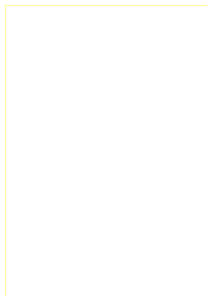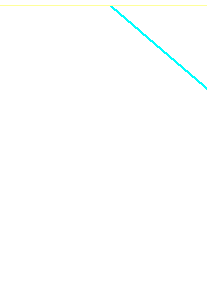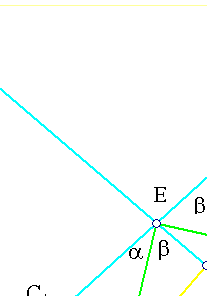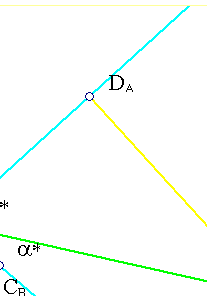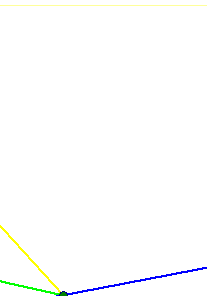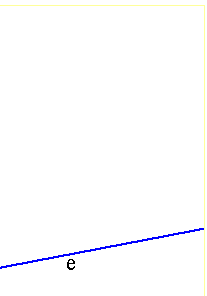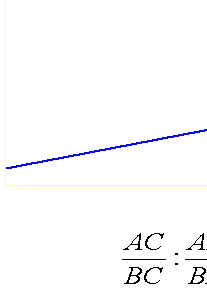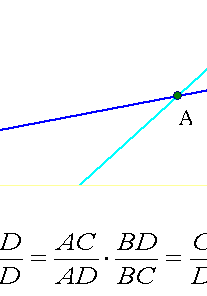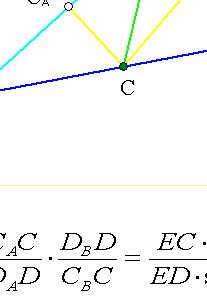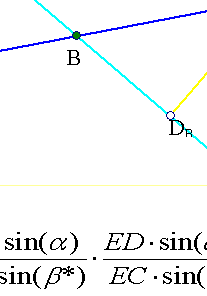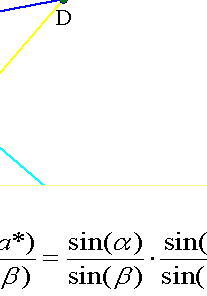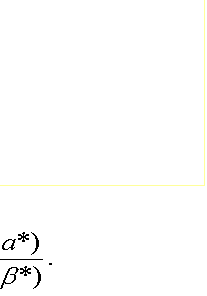##2. Cross ratio coincurence criteria

The following two properties related to the cross ratio of a pencil of lines E(ABCD) have important applications.

(I) If two lines e, e' carry each four points with the same cross ratio, (ABCD)=(A'B'C'D') say, and two corresponding points coincide      (B=B' say i.e. e, e' intersect at B=B'). Then the lines joining corresponding points pass through the same point E.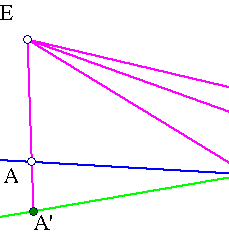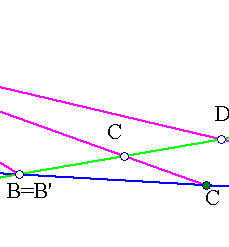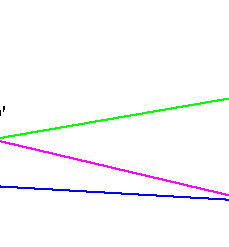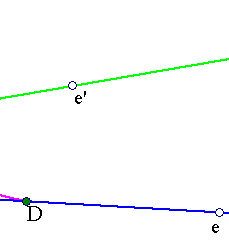(II) If two line pencils E(ABCD), E'(A'B'C'D') have a common line (f.e. EC = E'C') then the other pairs of homologous lines intersect at three collinear points.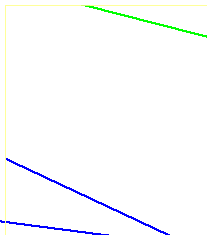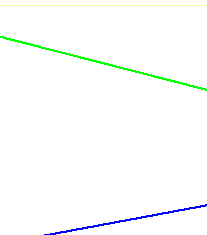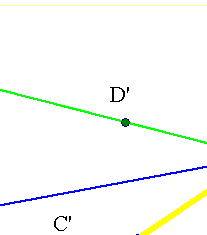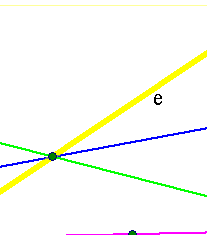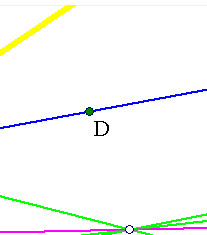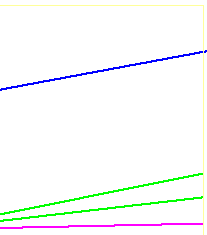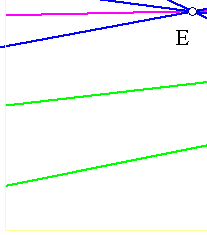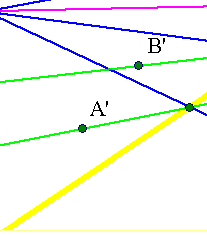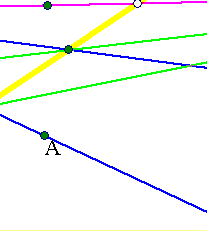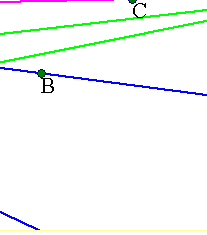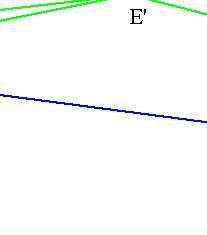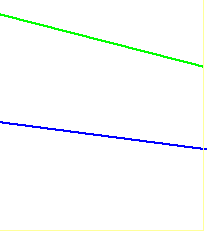The two propositions are dual to each other and trivial to prove. Consider f.e. in the second property line e of the intersection points of pairs (EA, E'A') and (EB, E'B'). Then by the invariance of the cross ratio on e, the intersection point of e with ED must coincide with that of E'D'. First property can serve as basis for a simple proof of Pascal's theorem on hexagons inscribed in conics (see Pascal.html ).

##3. Harmonic pencils (or bundles) of lines

A pencil of four lines through a point E, E(A,B,C,D) is called harmonic if the cross ratio of the four lines (in that order) is -1.
One prominent example of harmonic pencil consists of an angle and its two bisectors.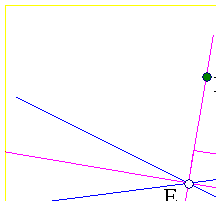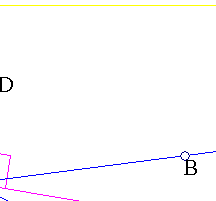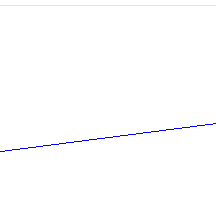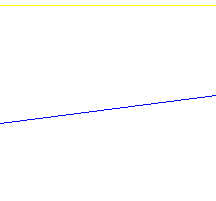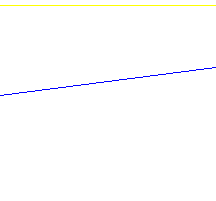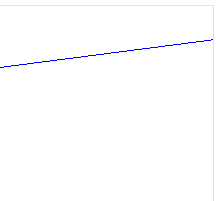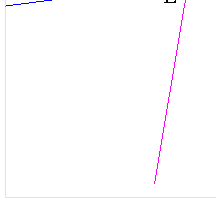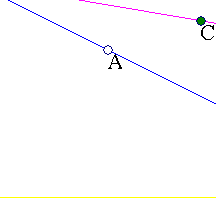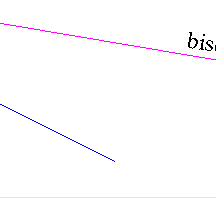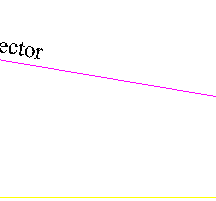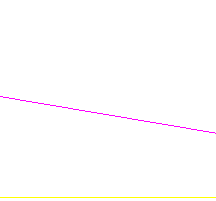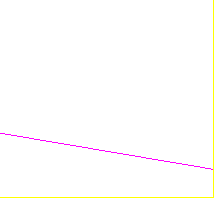Another prominent example is the one related to a complete quadrilateral ABCD. This is created by fixing four points in general position. It has six sides resulting by all combinations of the points by two. It defines also three additional points (below labeled by {E,F,G}) named diagonal points. The three lines through the diagonal points are called diagonals. At every diagonal point the two concurring sides and two diagonals build a harmonic pencil. Thus, in the example below F(A,B,G,E), E(A,D,F,G), G(C,D,F,E) are harmonic pencils. The reason for that can be found in Harmonic.html .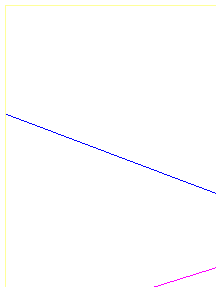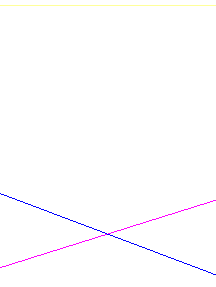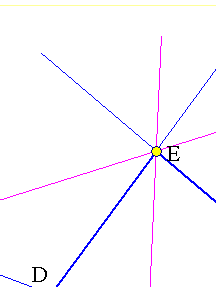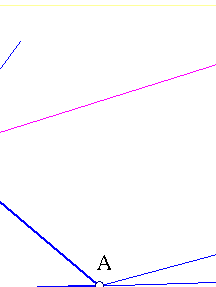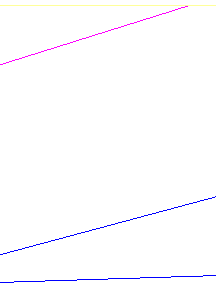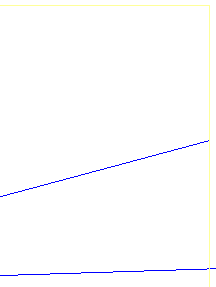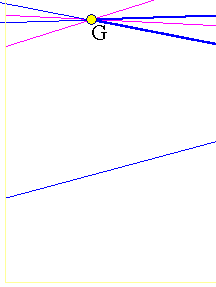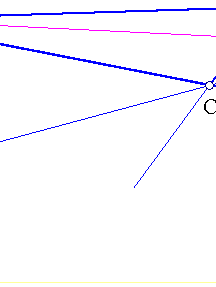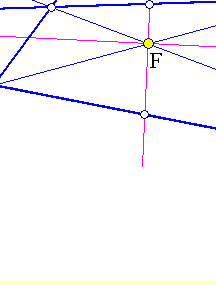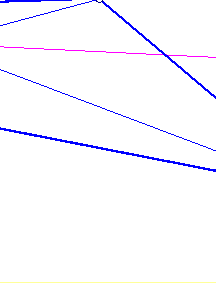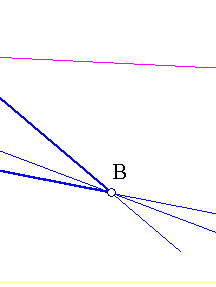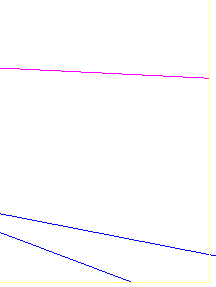A third example of harmonic pencil of four lines is the one formed by two sides {AB,AC} of a triangle ABC, the correspondingmedian AD and the corresponding parallel AE to the basis BC. This and other examples of harmonic pencils are examined in the file Harmonic_Bundle.html .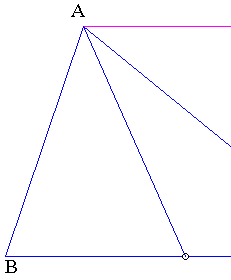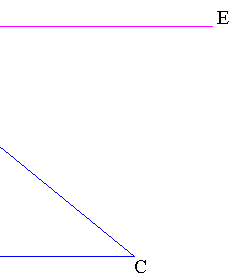##4. Harmonic conjugation of lines

Given the four lines through the origin in the form {ai x + bi y = 0, i=1,..,4}, their cross ratio can be seen to be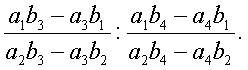In particular, if the lines are given in the form { y = ai x, i=1,..,4}, then their cross ratio is given by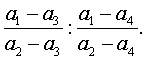In particular, if the lines make a harmonic pencil, then last ratio is -1. There results an interesting transformation each time one isgiven two fixed lines, say determined by their corresponding coefficients {a1, a2}. The transformation is called harmonic conjugation with respect to the two given lines and corresponds to each other line y = a3x, the unique fourth line y = a4x, such that the four lines make a harmonic pencil. Equating the above ratio to -1 and solving for a4 (in terms of a3) we find the equation.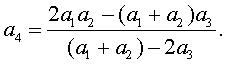This is an involutive Moebius transformation (see Involution.html )  whose determinant is equal to (a1-a2)2, which is non-zero if thetwo initial lines are different. Thus, the harmonic conjugation with respect to the two initial lines is described by an involutive Moebius transformation of the line-coefficients, the Moebius transformation in turn being determined by the coefficients of the two given lines.